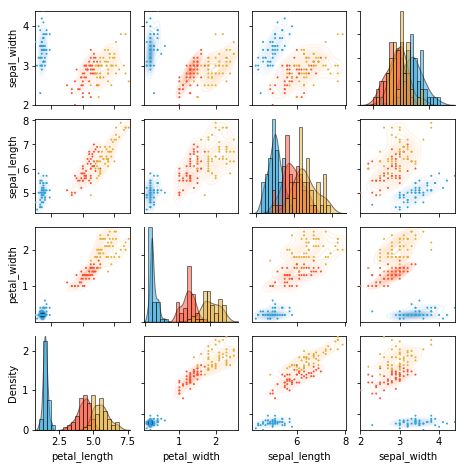# Iris grouped grid¶

Most examples work across multiple plotting backends, this example is also available for:

In :
import holoviews as hv
from holoviews import opts

hv.extension('matplotlib')## Declaring data¶

In :
from bokeh.sampledata.iris import flowers
from holoviews.operation import gridmatrix

iris_ds = hv.Dataset(flowers).groupby('species').overlay()


## Plot¶

In :
density_grid = gridmatrix(iris_ds, diagonal_type=hv.Distribution, chart_type=hv.Bivariate)
point_grid = gridmatrix(iris_ds, chart_type=hv.Points)

(density_grid * point_grid).opts(
opts.Bivariate(bandwidth=0.5, cmap=hv.Cycle(values=['Blues', 'Reds', 'Oranges'])),
opts.Histogram(alpha=0.5), opts.Points(s=4))

Out: